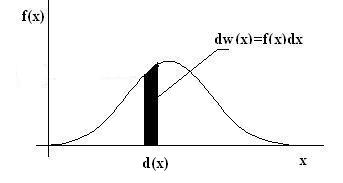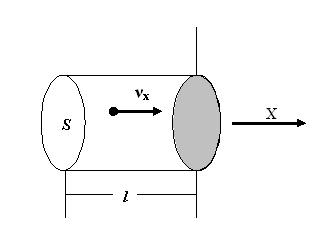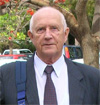# Ideal Gases

We deal with gases every day even if we don't think about it, just because we are breathing. The ambient gas of our atmosphere, primarily composed of nitrogen and oxygen, enters our lungs where oxygen is adsorbed for various chemical reactions which support our life. It happens every moment, day and night, and it happens now when you read this page.

What is the difference between a real and an ideal gas? As usual, physics deals with some simplifications (approximations), which allow the use of the power of mathematics to create elegant formulas which have predictive power. Moreover, by making such simplifications, physicists tell also when and often why they would not work and what needs to be done beyond the boundaries of these simplifications in order to describe the real world phenomena. The gases are made of atoms and molecules which interact with each other at short distances, however, the ideal gas approximation is based on an assumption that these interactions, as well as the volume of the molecules, can be ignored. Moreover, gaseous molecules are in constant random motion and exchange energy in elastic collisions (another approximation!) among themselves and within the walls.

In many ways, molecules of ideal gases behave like billiard balls, but the physics of ideal gases are not dealing with each individual molecule, like Newton's physics of classical particles but rather with their statistical ensembles, made of an enormously large number of molecules. Physics of gases is based on the laws of statistical thermodynamics. The behavior of each individual molecule is described by probabilities for it to be at a certain point of space and move in some specific direction with a specified speed (momentum).

The ideal gases as are described by a restricted set of parameters unified by corresponding equations of state. The parameters describing such gases comprise mass (M), pressure (p), density (ρ), temperature (T), and volume (V). Any statistical system can be in various states described by a set of parameters. These parameters do not always have certain values. If, for example, pressure (or temperature) in different elementary volumes of gas is different, it is impossible to attribute certain general values to the whole gas. Such a state is referred to as a nonequilibrium state. If such a nonequilibrium closed system is left alone for a period of time, the temperature and/or pressure inside the system will equalize in due course. The system will have passed into a state of equilibrium.

## Distribution Function

For the definition of the average value of any characteristic of an ideal gas, statistical physics offers a special function, the so-called function of distribution of particles for a given physical property. This distribution is not uniform; for example, the concentration of molecules with low speed is much less than the concentration of molecules with average speed. Let's designate by x a value of distribution upon which a system of N molecules is examined; this can be speed, energy, etc. We shall designate dN(x) as the number of particles, the parameter x of which lies in a limit from x up to x + dx, dN(x) = f(x)Ndx, where f(x)= dN/Ndx is a distribution function for x property. The average value of the x property can be calculated by the formula <x> = ∫xf(x)dx. The figure below presents an example of a distribution function:## General equation of an ideal gas

One of the basic features of gas is its property to exert pressure on the walls of the vessel in which it is contained. Let us determine this pressure for a state of ideal gas. On the way, we shall obtain a number of other important equations. In spite of the fact that we will be using a considerably simplified model, we will obtain results conterminous to our exact consideration.

In addition to those simplifications that we have already made when introducing the ideal gas model, we will also make some additional assumptions that will help us to simplify our calculations. In particular, we will: (1) count all molecules as identical, spherically symmetric and moving at the same speeds (let this speed be the average root square velocity vrms = √<v²>); (2) consider that all molecules move with equal probability along three coordinate axes, i.e., 1/6 part of all molecules moves along each direction.

Let's choose a cylindrical element with the cross–section S and a side l in the vessel close to the wall. Each molecule hitting the wall obtains from it a momentum Δp = -2mv. In turn, the molecule transfers the momentum Δp = 2mv to the vessel's wall. Assuming a molecule–wall interaction time to be equal τ we can obtain the force produced by this impact F = 2Δmvrms/τ, and acting on the wall by a single molecule. In order to find the total force, we need to find the number of molecules that hit the wall in time τ. If we choose a cylinder of length equal to l = vrmsτ (see figure below), then all molecules in the cylinder moving toward the wall hit the wall; their number is (1/6)n×2mvrmsτ. Then the force exerted upon the wall from the molecules in the cylinder will be Fp = (1/6)nSvrmsτ(2mv²rmsτ/τ) = (1/3)nSv²rmsm, and the pressure will be p = F/S = (2/3)n(mv²rms/2).Taking into account the fact that the actual speed of all the molecules vary, we should take the average values of the speed squared, <ε> = <mv²/2>, and the pressure, p = (2/3)n<ε>. Therefore, the pressure acquired by the ideal gas on the vessel's walls is equal to 2/3 of the volumetric density of the translational kinetic energy of the molecules.

p = (2/3)n(mv²/2)

Since n<ε> = N<ε>/V = K/V = w, where w is the volume averaged kinetic energy density. Therefore, the pressure acquired by the ideal gas on the vessel's walls is equal to 2/3 of the volumetric density of the translational kinetic energy.

Another form of this equation can be obtained by its multiplication with volume in moles

pVm = (2/3)nVm<ε>

Since pVm=RT and nVm=NA, then RT=(2/3)NA<ε>. The ratio (R/NA)=k is referred to as the Boltzmann constant (1.38x10-23 J/K). Then,

<ε> = (3/2)kT.

i.e., the average kinetic energy of the translational energy of the molecules of the ideal gas is equal to 3/2 of the product of the Boltzmann constant and absolute temperature. Therefore, product kT is a measure of the thermal movement of molecules.

It follows from the last formula that absolute temperature T is a measure of the average kinetic energy of the translational motion of molecules. Two important conclusions follow from this: at zero absolute temperature the translational kinetic energy of each molecule is zero; their motion stops. The second conclusion is that the ideal gas temperature can be measured in energy units. In reality, at a room temperature of 300 K, the averaged value of energy <ε> is 1.38x10-23x300 J=4x10-21 J; it is equivalent to saying “the gas energy is 300 K” or “the gas temperature is 25 meV”.

There exists a relation between the pressure and absolute temperature. It can be obtained from comparison of eqs. (3.1.11) and (3.1.12). We can obtain

p=nkT

Multiplying this equation by molecule mass m we can obtain the expression,

T = (mp/kρ)

Physics of ideal gas is simple, elegant and has a lot of predictive power!

## AcknowledgementThis web page is created by professor Ruslan Ozerov, who is retired from D. Mendeleyev University of Chemical Technology of Russia and lives in Perth, Australia.